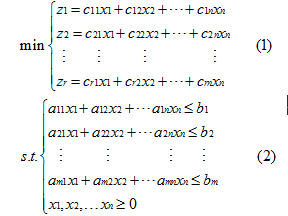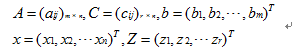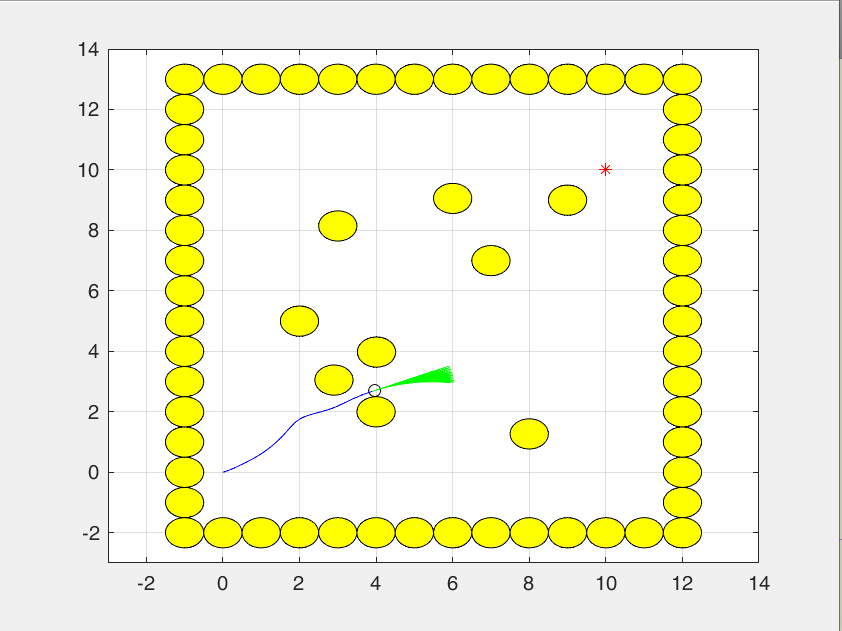• 线性规划.非线性规划.多目标规划Matlab代码[比赛已经用过,保真好使]
• 多目标规划matlab程序实现，word版本，论文格式。很强大！
• 粒子群算法 约束多目标 优化 matlab代码 粒子群算法 约束多目标 优化 matlab代码 粒子群算法 约束多目标 优化 matlab代码 粒子群算法 约束多目标 优化 matlab代码
• 关于多目标选址规划问题的flod算法matlab代码实现
• 关于多目标选址规划问题的flod算法matlab代码实现。
• 最新粒子群算法求解约束多目标优化万能matlab代码。 粒子群算法 约束多目标 优化 matlab代码 粒子群算法 约束多目标 优化 matlab代码
• 多点路径规划指标欢迎使用杠杆.js-活细胞和细胞器显微镜的计算工具 everlejs旨在直接从显微镜读取数据，并具有即时分析和可视化功能，可显示通道3D和2D缩时图像数据。 观看实际操作。 单击即可启动您自己的服务器...
• 偶写的一个关于目标规划的M文件，利用fgoalattain实现多目标规划，并实现对文本文件的读写功能。
• 突变-在目标文件的源代码中更改某些语句。 Mutator-一种自动在编程文件上进行突变的软件工具。 Mutationdocker-配置为运行mutator的虚拟机环境。 突变-更改了与原始程序不同代码的编程文件。 关于 网络物理突变工具...
• ## 整数规划Matlab代码

千次阅读 2019-08-20 19:48:54
%整数规划代码 f = [1;1;1;1;1;1;1;1;1;1;1;1;1]; A= [-13 -12 -11 -10 -9 -8 -7 -6 -5 -4 -3 -2 -1; -3 -4 -4 -4 -4 -4 -3 -3 -3 -4 -4 -4 -4 ; -6 -5 -5 -5 -5 -5 -6 -6 -6 -5 -5 -5 -5 ; -12 -12 -12 -13 -13 -13...
%整数规划代码
f = [1;1;1;1;1;1;1;1;1;1;1;1;1];
A= [-13 -12 -11 -10 -9 -8 -7 -6 -5 -4 -3 -2 -1;
-3 -4 -4 -4 -4 -4 -3 -3 -3 -4 -4 -4 -4 ;
-6 -5 -5 -5 -5 -5 -6 -6 -6 -5 -5 -5 -5 ;
-12 -12 -12 -13 -13 -13 -12 -12 -12 -12 -12 -13 -13 ;
-4 -4 -4 -3 -3 -3 -4 -4 -4 -4 -4 -3 -3;
-5 -5 -5 -5 -6 -6 -5 -6 -5 -5 -5 -5 -6 ;
-13 -13 -13 -13 -12 -12 -12 -12 -13 -13 -13 -13 -12;
-3 -3 -3 -3 -4 -4 -4 -4 -3 -3 -3 -3 -4;
-5 -6 -6 -6 -5 -5 -5 -5 -5 -6 -6 -6 -5 ;
-13 -12 -12 -12 -13 -12 -13 -13 -13 -12 -12 -12 -13;
-3 -4 -4 -4 -3 -4 -3 -3 -3 -4 -4 -4 -13;
-6 -5 -5 -5 -5 -5 -6 -6 -6 -5 -5 -5 -5 ;
-12 -13 -13 -13 -13 -13 -12 -12 -12 -13 -13 -13 -13;
-4 -3 -3 -3 -3 -3 -4 -4 -4 -3 -3 -3 -3 ;
-5 -5 -5 -5 -6 -6 -5 -5 -5 -5 -5 -5 -6 ;
-13 -13 -13 -13 -12 -12 -13 -13 -13 -13 -13 -13 -12;
-3 -3 -3 -3 -4 -4 -3 -3 -3 -3 -3 -3 -4;
-5 -6 -6 -6 -5 -5 -5 -5 -5 -6 -6 -6 -5;
-13 -12 -12 -12 -13 -13 -13 -13 -13 -12 -12 -12 -13 ;
-3 -4 -4 -4 -3 -3 -3 -3 -3 -4 -4 -4 -3 ;
-6 -5 -5 -5 -5 -5 -6 -6 -6 -5 -5 -5 -5 ;
-1 0 0 0 0 0 0 0 0 0 0 0 0 ;
0 -1 0 0 0 0 0 0 0 0 0 0 0 ;
0 0 -1 0 0 0 0 0 0 0 0 0 0 ;
0 0 0 -1 0 0 0 0 0 0 0 0 0 ;
0 0 0 0 -1 0 0 0 0 0 0 0 0 ;
0 0 0 0 0 -1 0 0 0 0 0 0 0 ;
0 0 0 0 0 0 -1 0 0 0 0 0 0 ;
0 0 0 0 0 0 0 -1 0 0 0 0 0 ;
0 0 0 0 0 0 0 0 -1 0 0 0 0 ;
0 0 0 0 0 0 0 0 0 -1 0 0 0 ;
0 0 0 0 0 0 0 0 0 0 -1 0 0 ;
0 0 0 0 0 0 0 0 0 0 0 -1 0 ;
0 0 0 0 0 0 0 0 0 0 0 0 -1 ];
b = [-186.73;-62.56;-81;-186.73;-62.56;-81;-186.73;-62.56;-81;-186.73;-62.56;-81;-186.73;-62.56;-81;-186.73;-62.56;-81;-186.73;-62.56;-81;0;0;0;0;0;0;0;0;0;0;0;0;0];
C=[];
d=[];
xm=[0;0];
xM=1e+10*[1;1];
x0=[0;0];
%intlinprog 函数,用于进行整数规划和整数非整数的混合规划
[x,y,flag]=intlinprog(f,[1:13],A,b,C,d,xm,xM)

其中intlinprog函数中： [x,fval,exitflag,output] =intlinprog(f,intcon,A,b,Aeq,beq,lb,[]) intcon是整数变量地址，是重要参数。
展开全文数学建模
• 多点路径规划指标光环UAS AuraUAS是一个完整的，自包含的自动驾驶生态系统。 它不是从任何其他流行的diy自动驾驶仪系统派生的，而是具有独立的开发历史的。 硬件架构 Teensy（arduino / teensyduino）处理器，用于...
• 求解目标线性规划的基本思想是将目标转化为单目标，常见的方法有理想点法、线性加权法、最大最小法、目标规划法、模糊数学解法等。这里就这几种方法进行举例说明，并用matlab实现。 一、目标线性规划模型 ...
求解多目标线性规划的基本思想是将多目标转化为单目标，常见的方法有理想点法、线性加权法、最大最小法、目标规划法、模糊数学解法等。这里就这几种方法进行举例说明，并用matlab实现。

一、多目标线性规划模型
多目标线性规划模型是有两个或两个以上的目标函数，且所有的目标函数和约束条件都是线性的，数学模型表示为：我们记则上述目标规划可简化为：

展开全文• 有各类多目标规划问题，和相应得到求解理论方法，还有对应的matlab程序
• % ------------------------------------------------------------------------- % % File : DWA % ...% Environment : Matlab % % Author :Yuncheng Jiang % % % License : Modified BSD Softwar.% -------------------------------------------------------------------------
%
% File : DWA
%
% Discription : Mobile Robot Motion Planning with Dynamic Window Approach
%
% Environment : Matlab
%
% Author :Sam
%
%
% log: collision checking 修改为硬约束
% -------------------------------------------------------------------------

function [] = dwa_V_1_0()

close all;
clear ;

disp('Dynamic Window Approach sample program start!!')

% x=[0 0 pi/2 0 0]'; % 5x1矩阵 列矩阵  位置 0，0 航向 pi/2 ,速度、角速度均为0
x = [0 0 pi/10 0 0]';

POSE_X      = 1;  %坐标 X
POSE_Y      = 2;  %坐标 Y
YAW_ANGLE   = 3;  %机器人航向角
V_SPD       = 4;  %机器人速度
W_ANGLE_SPD = 5;  %机器人角速度

goal = [10,10];   % 目标点位置 [x(m),y(m)]

% 障碍物位置列表 [x(m) y(m)]

obstacle=[%0 2;
3 10*rand(1);
%           4 4;
%          5 4;
%            5 5;
6 10*rand(1);
%          5 9
%          7 8
8 10*rand(1);
2 5;
4 2;
7 7;
9 9
];
%边界障碍物，防止跑出图外
for i =-1
for j = -1:12
obstacle = [obstacle; [i,j]];
end
end
for i =12
for j = -1:12
obstacle = [obstacle; [i,j]];
end
end
for j =-2
for i = -1:12
obstacle = [obstacle; [i,j]];
end
end
for j=13
for i= -1:12
obstacle = [obstacle; [i,j]];
end
end

obstacleR = 0.5;% 冲突判定用的障碍物半径
global dt;
dt = 0.1;% 时间[s]

% 机器人运动学模型参数
%定义Kinematic的下标含义
MD_MAX_V    = 1;%   最高速度m/s]
MD_ACC      = 3;%   加速度[m/ss]
MD_V_RESOLUTION  = 5;%  速度分辨率[m/s]

% 航向得分的比重、距离得分的比重、速度得分的比重、向前模拟轨迹的时间
evalParam = [0.045, 0.1 ,0.1, 3.0];
% evalParam = [2, 0.2 ,0.2, 3.0];
area      = [-3 14 -3 14];% 模拟区域范围 [xmin xmax ymin ymax]

% 模拟实验的结果
result.x=[];   %累积存储走过的轨迹点的状态值
tic; % 估算程序运行时间开始
flag_obstacle = [1-2*rand(1) 1-2*rand(1) 1-2*rand(1)];
vel_obstacle = 0.05;
temp = 0;
abc = 0;
%movcount=0;
%% Main loop   循环运行 5000次 指导达到目的地 或者 5000次运行结束
for i = 1:5000
if mod(i,50)==0
end
% DWA参数输入 返回控制量 u = [v(m/s),w(rad/s)] 和 轨迹
[u,traj] = DynamicWindowApproach(x,Kinematic,goal,evalParam,obstacle,obstacleR);%算出下发速度u/当前速度u
x = f(x,u);% 机器人移动到下一个时刻的状态量 根据当前速度和角速度推导 下一刻的位置和角度
abc = abc+1;
% 历史轨迹的保存
result.x = [result.x; x'];  %最新结果 以行的形式 添加到result.x，保存的是所有状态参数值，包括坐标xy、朝向、线速度、角速度，其实应该是只取坐标就OK

% 是否到达目的地
if norm(x(POSE_X:POSE_Y)-goal')<0.25   % norm函数来求得坐标上的两个点之间的距离
disp('==========Arrive Goal!!==========');break;
end

%====Animation====
hold off;               % 关闭图形保持功能。 新图出现时，取消原图的显示。
ArrowLength = 0.5;      % 箭头长度

% 机器人
% quiver(x,y,u,v) 在 x 和 y 中每个对应元素对组所指定的坐标处将向量绘制为箭头
quiver(x(POSE_X), x(POSE_Y), ArrowLength*cos(x(YAW_ANGLE)), ArrowLength*sin(x(YAW_ANGLE)),'ok');
% 绘制机器人当前位置的航向箭头
hold on;
%启动图形保持功能，当前坐标轴和图形都将保持，从此绘制的图形都将添加在这个图形的基础上，并自动调整坐标轴的范围

plot(result.x(:,POSE_X),result.x(:,POSE_Y),'-b');hold on;    % 绘制走过的所有位置 所有历史数据的 X、Y坐标
plot(goal(1),goal(2),'*r');hold on;                          % 绘制目标位置

for j = 1:3
if obstacle(j,2) > 10 && flag_obstacle(j) > 0 || obstacle(j,2) < 0 && flag_obstacle(j) < 0
flag_obstacle(j) = -flag_obstacle(j);
end
%        obstacle(j,2)=obstacle(j,2)+flag_obstacle(j)*vel_obstacle;
end

%plot(obstacle(:,1),obstacle(:,2),'*k');hold on;              % 绘制所有障碍物位置
DrawObstacle_plot(obstacle,obstacleR);

% 探索轨迹 画出待评价的轨迹
if ~isempty(traj) %轨迹非空
for it=1:length(traj(:,1))/5    %计算所有轨迹数  traj 每5行数据 表示一条轨迹点
ind = 1+(it-1)*5; %第 it 条轨迹对应在traj中的下标
plot(traj(ind,:),traj(ind+1,:),'-g');hold on;  %根据一条轨迹的点串画出轨迹   traj(ind,:) 表示第ind条轨迹的所有x坐标值  traj(ind+1,:)表示第ind条轨迹的所有y坐标值
end
end

axis(area); %根据area设置当前图形的坐标范围，分别为x轴的最小、最大值，y轴的最小最大值
grid on;
drawnow limitrate;  %刷新屏幕. 当代码执行时间长，需要反复执行plot时，Matlab程序不会马上把图像画到figure上，这时，要想实时看到图像的每一步变化情况，需要使用这个语句。
for j = 1:3
if norm(obstacle(j,:)-x(1:2)')-obstacleR < 0
disp('==========Hit an obstacle!!==========');
temp = 1;
break;
end
end
if temp == 1
break;
end
% movcount = movcount+1;
% mov(movcount) = getframe(gcf);%  记录动画帧
end
toc;  %输出程序运行时间  形式：时间已过 ** 秒。
disp(abc)
%VideoWriter(mov,'movie.avi');  %录制过程动画 保存为 movie.avi 文件

%% 绘制所有障碍物位置
% 输入参数：obstacle 所有障碍物的坐标   obstacleR 障碍物的半径
function [] = DrawObstacle_plot(obstacle,obstacleR)
r = obstacleR;
theta = 0:pi/20:2*pi;
for id=1:length(obstacle(:,1))
x = r * cos(theta) + obstacle(id,1);
y = r  *sin(theta) + obstacle(id,2);
%plot(x,y,'-m');
fill(x,y,'y');
end
%plot(obstacle(:,1),obstacle(:,2),'*m');hold on;              % 绘制所有障碍物位置

%% DWA算法实现
% 输入参数：当前状态、模型参数、目标点、评价函数的参数、障碍物位置、障碍物半径
% 返回参数：控制量 u = [v(m/s),w(rad/s)] 和 轨迹集合 N * 31  （N：可用的轨迹数）
% 选取最优参数的物理意义：在局部导航过程中，使得机器人避开障碍物，朝着目标以较快的速度行驶。
function [u,trajDB] = DynamicWindowApproach(x,model,goal,evalParam,ob,R)
% Dynamic Window [vmin,vmax,wmin,wmax] 最小速度 最大速度 最小角速度 最大角速度速度
Vr = CalcDynamicWindow(x,model);  % 根据当前状态 和 运动模型 计算当前的参数允许范围

% 评价函数的计算 evalDB N*5  每行一组可用参数 分别为 速度、角速度、航向得分、距离得分、速度得分
%               trajDB      每5行一条轨迹 每条轨迹都有状态x点串组成

if isempty(evalDB)
disp('no path to goal!!');
u=[0;0];return;
end

% 各评价函数正则化
evalDB = NormalizeEval(evalDB);

% 最终评价函数的计算
feval=[];
for id=1:length(evalDB(:,1))
feval = [feval;evalParam(1:3)*evalDB(id,3:5)']; %根据评价函数参数 前三个参数分配的权重 计算每一组可用的路径参数信息的得分
end
evalDB = [evalDB feval]; % 最后一组；加最后一列，每一组速度的最终得分

[maxv,ind] = max(feval);% 选取评分最高的参数 对应分数返回给 maxv  对应下标返回给 ind
u = evalDB(ind,1:2)';% 返回最优参数的速度、角速度

%% 评价函数 内部负责产生可用轨迹
% 输入参数 ：当前状态、参数允许范围（窗口）、目标点、障碍物位置、障碍物半径、评价函数的参数
% 返回参数：
%           evalDB N*5  每行一组可用参数 分别为 速度、角速度、航向得分、距离得分、速度得分
%           trajDB      每5行一条轨迹 每条轨迹包含 前向预测时间/dt + 1 = 31 个轨迹点（见生成轨迹函数）
function [evalDB,trajDB] = Evaluation(x,Vr,goal,ob,R,model,evalParam)
evalDB = [];
trajDB = [];
for vt = Vr(1):model(5):Vr(2)       %根据速度分辨率遍历所有可用速度： 最小速度和最大速度 之间 速度分辨率 递增
for ot=Vr(3):model(6):Vr(4)     %根据角度分辨率遍历所有可用角速度： 最小角速度和最大角速度 之间 角度分辨率 递增
% 轨迹推测; 得到 xt: 机器人向前运动后的预测位姿; traj: 当前时刻 到 预测时刻之间的轨迹（由轨迹点组成）
[xt,traj] = GenerateTrajectory(x,vt,ot,evalParam(4));  %evalParam(4),前向模拟时间;
% 各评价函数的计算
[dist,Flag] = CalcDistEval(xt,ob,R);    % 前项预测终点 距离最近障碍物的间隙得分 距离越远分数越高
vel     = abs(vt);                  % 速度得分 速度越快分越高
stopDist = CalcBreakingDist(vel,model); % 制动距离的计算
if dist > stopDist && Flag == 0 % 如果可能撞到最近的障碍物 则舍弃此路径 （到最近障碍物的距离 大于 刹车距离 才取用）
evalDB = [evalDB;[vt ot heading dist vel]];
trajDB = [trajDB;traj];   % 每5行 一条轨迹
end
end
end

%% 归一化处理
% 每一条轨迹的单项得分除以本项所有分数和
function EvalDB=NormalizeEval(EvalDB)
% 评价函数正则化
if sum(EvalDB(:,3))~= 0
EvalDB(:,3) = EvalDB(:,3)/sum(EvalDB(:,3));  %矩阵的数除  单列矩阵的每元素分别除以本列所有数据的和
end
if sum(EvalDB(:,4))~= 0
EvalDB(:,4) = EvalDB(:,4)/sum(EvalDB(:,4));
end
if sum(EvalDB(:,5))~= 0
EvalDB(:,5) = EvalDB(:,5)/sum(EvalDB(:,5));
end

%% 单条轨迹生成、轨迹推演函数
% 输入参数： 当前状态、vt当前速度、ot角速度、evaldt 前向模拟时间、机器人模型参数（没用到）
% 返回参数;
%           x   : 机器人模拟时间内向前运动 预测的终点位姿(状态);
%           traj: 当前时刻 到 预测时刻之间 过程中的位姿记录（状态记录） 当前模拟的轨迹
%                  轨迹点的个数为 evaldt / dt + 1 = 3.0 / 0.1 + 1 = 31
%
function [x,traj] = GenerateTrajectory(x,vt,ot,evaldt)
global dt;
time = 0;
u = [vt;ot];% 输入值
traj = x;   % 机器人轨迹
while time <= evaldt
time = time+dt; % 时间更新
x = f(x,u);     % 运动更新 前项模拟时间内 速度、角速度恒定
traj = [traj x]; % 每一列代表一个轨迹点 一列一列的添加
end

%% 计算制动距离
%根据运动学模型计算制动距离, 也可以考虑成走一段段圆弧的累积 简化可以当一段段小直线的累积
function stopDist = CalcBreakingDist(vel,model)
global dt;
MD_ACC   = 3;%
stopDist=0;
while vel>0   %给定加速度的条件下 速度减到0所走的距离
stopDist = stopDist + vel*dt;% 制动距离的计算
vel = vel - model(MD_ACC)*dt;%
end

%% 障碍物距离评价函数  （机器人在当前轨迹上与最近的障碍物之间的距离，如果没有障碍物则设定一个常数）
% 输入参数：位姿、所有障碍物位置、障碍物半径
% 输出参数：当前预测的轨迹终点的位姿距离所有障碍物中最近的障碍物的距离 如果大于设定的最大值则等于最大值
% 距离障碍物距离越近分数越低
function [dist,Flag] = CalcDistEval(x,ob,R)
dist=100;
for io = 1:length(ob(:,1))
disttmp = norm(ob(io,:)-x(1:2)')-R; %到第io个障碍物的距离 - 障碍物半径  ！！！有可能出现负值吗
if disttmp <0
Flag = 1;
break;
else
Flag = 0;
end

if dist > disttmp   % 大于最小值 则选择最小值
dist = disttmp;
end
end

% 障碍物距离评价限定一个最大值，如果不设定，一旦一条轨迹没有障碍物，将太占比重
if dist >= 3*R %最大分数限制
dist = 3*R;
end

% 输入参数：当前位置、目标位置
% 输出参数：航向参数得分  当前车的航向和相对于目标点的航向 偏离程度越小 分数越高 最大180分
theta = toDegree(x(3));% 机器人朝向
goalTheta = toDegree(atan2(goal(2)-x(2),goal(1)-x(1)));% 目标点相对于机器人本身的方位
if goalTheta > theta
targetTheta = goalTheta-theta;% [deg]
else
targetTheta = theta-goalTheta;% [deg]
end

%% 计算动态窗口
% 返回 最小速度 最大速度 最小角速度 最大角速度速度
function Vr = CalcDynamicWindow(x,model)

V_SPD       = 4;%机器人速度
W_ANGLE_SPD = 5;%机器人角速度

MD_MAX_V = 1;%
MD_MAX_W = 2;%
MD_ACC   = 3;%
MD_VW    = 4;%

global dt;
% 车子速度的最大最小范围 依次为：最小速度 最大速度 最小角速度 最大角速度速度
Vs=[0 model(MD_MAX_V) -model(MD_MAX_W) model(MD_MAX_W)];

% 根据当前速度以及加速度限制计算的动态窗口  依次为：最小速度 最大速度 最小角速度 最大角速度速度
Vd = [x(V_SPD)-model(MD_ACC)*dt x(V_SPD)+model(MD_ACC)*dt ...
x(W_ANGLE_SPD)-model(MD_VW)*dt x(W_ANGLE_SPD)+model(MD_VW)*dt];

% 最终的Dynamic Window
Vtmp = [Vs;Vd];  %2 X 4  每一列依次为：最小速度 最大速度 最小角速度 最大角速度速度
Vr = [max(Vtmp(:,1)) min(Vtmp(:,2)) max(Vtmp(:,3)) min(Vtmp(:,4))];

%% Motion Model 根据当前状态推算下一个控制周期（dt）的状态
function x = f(x, u)
global dt;
F = [1 0 0 0 0
0 1 0 0 0
0 0 1 0 0
0 0 0 0 0
0 0 0 0 0];

B = [dt*cos(x(3)) 0
dt*sin(x(3)) 0
0 dt
1 0
0 1];

x= F*x+B*u;

%% END


展开全文• 突变-在目标文件的源代码中更改某些语句。 Mutator-一种自动在编程文件上进行突变的软件工具。 Mutationdocker-配置为运行mutator的虚拟机环境。 突变-更改了与原始程序不同代码的编程文件。 关于 网络物理突变工具...
• 多点路径规划指标cuOMT：自适应蒙特卡洛最优传输算法的CUDA / C ++实现 这项工作是根据许可的。 该存储库包含cuOMT ，这是一个使用自适应蒙特卡洛最优传输（MC-OT）算法的CUDA / C ++求解器。 请参阅以获取PyTorch...
• 多目标搜索算法相对于单目标算法来说，更加贴近于实际问题，求解结果更具有参考价值。通过多目标搜索算法最终得到的不是一个最优解，而是一个非劣解集，需要从非劣解集中根据实际问题的需要选择一个解作为该问题的...
• 求解多目标规划 1.理想点法 clc,clear; close all; f=[3,-2];A=[2,3;2,1];b=[18;10];lb=[0;0]; [x,fval]=linprog(f,A,b,[],[],lb) clc,clear; close all; f=[3,-2];A=[2,3;2,1];b=[18;10];lb=[0;0]; [x,fval]=...
求解多目标规划
1.理想点法
clc,clear;
close all;
f=[3,-2];A=[2,3;2,1];b=[18;10];lb=[0;0];
[x,fval]=linprog(f,A,b,[],[],lb)

clc,clear;
close all;
f=[3,-2];A=[2,3;2,1];b=[18;10];lb=[0;0];
[x,fval]=linprog(f,A,b,[],[],lb)

2.目标规划法
function f=fun(x)
f(1)=3*x(1)-2*x(2);
f(2)=-4*x(1)-3*x(2);
end

clc,clear;
close all;
goal=[18,10];weight=[18,10];x0=[1,1];A=[2,3;2,1];b=[18,10];lb=zeros(2,1);
[x,fval]=fgoalattain('fun',x0,goal,weight,A,b,[],[],lb,[])

3.最大最小法
clc,clear;
close all;
x0=[1;1];A=[2,3;2,1];b=[18,10];lb=zeros(2,1);
[x,fval]=fminimax('fun',x0,A,b,[],[],lb,[])

展开全文• 基于MATLAB多目标线性规划_理想点法求解程序代码
• 多目标微粒子群算法MOPSO MATLAB代码，及一些文章说明
• 介绍了求解多目标的几种基本的方法，并附有matlab的一些基本语句！！lambda 算法 function 优化
• 线性回归的MatLab例子（带有数据与代码，可运行显示图形）
• ## 路径规划A*算法matlab代码注释

万次阅读 多人点赞 2019-01-17 21:29:31
路径规划A*算法matlab代码注释 文中所使用的代码出自手把手教用matlab做无人驾驶（三）-路径规划A*算法 一、Aplanning.m disp('A Star Path Planing start!!') p.start=[1,1]; %起始点 p.goal=[3,5]; %目标点 ...A*算法
• ## 多目标线性规划及matlab应用

千次阅读 多人点赞 2020-03-27 15:55:22
4.多目标规划MATLAB求解 （1）理想点法 （2）线性加权和法 （3）最大最小法 在实际问题中，衡量一个设计方案的好坏往往不止一个标准，常常要考虑个目标。例如研究生产过程时，人们既要提高生产效率，同时还要...算法
• %启发式信息，取为至目标点的直线距离的倒数 %下面构造启发式信息矩阵 for i=1:N ix=a*(mod(i,MM)-0.5); if ix==-0.5 ix=MM-0.5; end iy=a*(MM+0.5-ceil(i/MM)); % for j=1:N % jx=a*(mod(j,MM)-0.5); % ...蚁群算法...

# 多目标规划matlab代码matlab 订阅